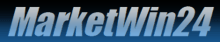Home | MarketWin24 \$B"M(B \$BFC@-(B \$B"N(B \$B>pJs%a%K%e!<(B \$B"N(B \$BG[?.%b%G%k\$H%7%9%F%`(B \$B"N(B \$B%5%s%W%k(B \$B"N(B MarketWin24+

 CONCEPT As an independent financial information consultant based in Tokyo, we gather and analyze information relating to Japan's economy, politics, and policy by utilizing our unique relationships, and provide market-oriented information from Japan to global investors. Making "Information for Performance" a principle, our goal is to contribute to subscribers' profitability.--\$B>pJs%a%K%e!<(B--

\$B0YBXAj>l8+DL\$7(B
 \$B"#(B \$B%G%\$%j!lM=B,(B \$BF|JA\$H6qBNE*\$JGdGc%]%\$%s%H\$r>\=R(B \$B;XI8\$d%\$%Y%s%H\$4\$H\$K;T>l\$NH?1~\$r;vA0%7%_%e%l!<%7%g%s(B \$BCxL>;T>l4X78M=B,(B [\$BD6C;4|M=B,(B] 1.\$BEl5~;T>l\$N%9%?!<%HA0\$K%U%!%s%@%a%s%?%k%:!"%F%/%K%+%kAPJ}\$G\$NM=B,(B 2.\$B%9%?!<%H8e\$N<{5k%*!<%@! 3.\$B@aL\%a%I!"%F%/%K%+%k!&%]%\$%s%H!"%9%H%C%W%m%9\$J\$I\$rB(;~>\Js(B [\$BDj4|%a%K%e!<(B] 1.\$B%9%?!<%H%l%Y%k!"CgCM8+DL\$7!"%9%H%C%W%m%9!"FC [\$B%F%/%K%+%kJ,@O(B] 1.\$B%U%#%\%J%C%A!"%]%\$%s%H!u%U%#%.%e%"!"0lL\6Q9UI=!"%"%9%H%m!"JQ2=F|\$J\$I(B \$B"((B\$B%F%/%K%+%kJ,@O\$N@lLg2q

\$B;T67B.Js(B
 \$B"#(B \$BEl5~8aA0!"El5~8a8e!"%m%s%I%s;T>l!"#N#Y;T>l\$KJ,\$1\$F;T67\$rAm3g(B \$B%W%l%\$%d!<\$NF0\$-!"Aj>l\$rF0\$+\$7\$?:`NA!";T>l\$N1=\$r4^\$a\$FLVMe(B \$BAj>lJQF0;~\$K\$O!"GX7J\$H:#8e\$N9TJ}\$rB(:B\$K%U%)%m!<(B

\$BMW?MH/8@(B
 \$B"#(B \$BMW?MH/8@\$NB.Js\$H;T>l\$X\$N1F6A\$N2r@b(B \$BFH<+J!&F|6d#O#B\$d@/<#2H\$J\$I!K(B \$BF?L>%3%s%U%#%G%s%7%c%k>pJs!J:bL3>J!&F|6d\$N8=Lr44It\$+\$i\$NFH<+>pJs!K(B \$B"((B\$B#M#O#F%&%)%C%A!"#B#O#J%&%)%C%A!"1JEDD.%&%)%C%A!"#F#R#B%&%)%C%A\$J\$I(B

\$B7P:Q;XI8(B
 \$B"#(B \$B%G%\$%j! \$B7P:Q;XI8\$NB.Js\$H;T>l\$X\$N1F6A2r@b(B

\$B
 \$B"#(B \$B;T>l4XO"\$N%K%e!<%9B.Js\$H%K%e!<%92r@b(B

\$B!!!!(B